# Test: Measures Of Central Tendency And Dispersion- 1

## 40 Questions MCQ Test Quantitative Aptitude for CA CPT | Test: Measures Of Central Tendency And Dispersion- 1

Description
Attempt Test: Measures Of Central Tendency And Dispersion- 1 | 40 questions in 40 minutes | Mock test for CA Foundation preparation | Free important questions MCQ to study Quantitative Aptitude for CA CPT for CA Foundation Exam | Download free PDF with solutions
QUESTION: 1

Solution:
QUESTION: 2

Solution:
QUESTION: 3

### When it comes to comparing two or more distributions we consider

Solution:
QUESTION: 4

Which one is difficult to compute?

Solution:
QUESTION: 5

Which one is an absolute measure of dispersion?

Solution:
QUESTION: 6

Which measure of dispersion is the quickest to compute?

Solution:
QUESTION: 7

Which measures of dispersions is not affected by the presence of extreme observations?

Solution:
QUESTION: 8

Which measure of dispersion is based on the absolute deviations only?

Solution:
QUESTION: 9

Which measure is based on only the central fifty percent of the observations?

Solution:
QUESTION: 10

Which measure of dispersion is based on all the observations?

Solution:
QUESTION: 11

The appropriate measure of dispersions for open – end classification is

Solution:
QUESTION: 12

The most commonly used measure of dispersion is

Solution:
QUESTION: 13

Which measure of dispersion has some desirable mathematical properties?

Solution:
QUESTION: 14

If the profits of a company remains the same for the last ten months, then the standard deviation of profits for these ten months would be ?

Solution:
QUESTION: 15

Which measure of dispersion is considered for finding a pooled measure of dispersion after combining several groups?

Solution:
QUESTION: 16

A shift of origin has no impact on

Solution:
QUESTION: 17

The range of 15, 12, 10, 9, 17, 20 is

Solution:
QUESTION: 18

The standard deviation of, 10, 16, 10, 16, 10, 10, 16, 16 is

Solution:
QUESTION: 19

For any two numbers SD is always

Solution:
QUESTION: 20

If all the observations are increased by 10, then

Solution:
QUESTION: 21

If all the observations are multiplied by 2, then

Solution:
QUESTION: 22

(Dirction 22 - 40) Write down the correct answers. Each question carries two marks.

Q. What is the coefficient of range for the following wages of 8 workers?

Rs.80, Rs.65, Rs.90, Rs.60, Rs.75, Rs.70, Rs.72, Rs.85.

Solution: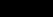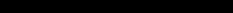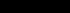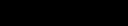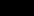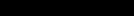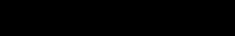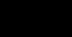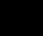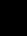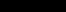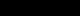QUESTION: 23

If Rx and Ry denote ranges of x and y respectively where x and y are related by 3x+2y+10=0, what would be the relation between x and y?

Solution:
QUESTION: 24

What is the coefficient of range for the following distribution?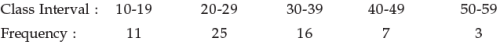Solution:
QUESTION: 25

If the range of x is 2, what would be the range of –3x +50?

Solution:

Explanation : Substituting x=2 in -3x+50

-3x+50 = -3(2)+50

= -6+50

= 44

QUESTION: 26

What is the value of mean deviation about mean for the following numbers?

5, 8, 6, 3, 4.

Solution:
QUESTION: 27

Median in set 6, 4, 2, 3, 4, 5, 5, 4 would be

Solution:
QUESTION: 28

The coefficient of mean deviation about mean for the first 9 natural numbers is

Solution:
QUESTION: 29

If the relation between x and y is 5y–3x = 10 and the mean deviation about mean for x is 12, then the mean deviation of y about mean is

Solution:

5y-3x=10
x=12
5y-3×12=10
5y-36=10
5y=46
y=46/5
y=9.2

QUESTION: 30

If two variables x and y are related by 2x + 3y –7 =0 and the mean and mean deviation about mean of x are 1 and 0.3 respectively, then the coefficient of mean deviation of y about mean is

Solution:
QUESTION: 31

The mean deviation about mode for the numbers 4/11,6/11,8/11,9/11,12/11,8/11 is 1/6?

Solution:
QUESTION: 32

If mean of percentages, rates and ratios is to be calculated then central tendency measure which must be used in this situation is

Solution:
QUESTION: 33

If the mean and SD of x are a and b respectively, then the SD of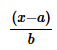is

Solution:
QUESTION: 34

What is the coefficient of variation of the following numbers?

53, 52, 61, 60, 64.

Solution:

The coefficient of variation of the following numbers  53,52,61,60,64​ are,
Step 1:
To find the mean of the above numbers,
x = 53+52+61+60+64 / 5 = 290/5 = 58
Step 2:
To find Standard deviation.,
S = √53² +52²+61²+60²+64² / 5  - (58²)
=√16390/5 -3364   = √3386-3364
=√22  = 4.69
Step 3 :
To find Coefficient of variation,
Coefficient of variation ( C. V) = ( S / X ) * 100
= ( 4.69 /58 ) * 100  = 0.0809 * 100
⇒8.09

QUESTION: 35

If the SD of x is 3, what us the variance of (5–2x)?

Solution:
QUESTION: 36

If x and y are related by 2x+3y+4 = 0 and SD of x is 6, then SD of y is

Solution:
QUESTION: 37

The quartiles of a variable are 45, 52 and 65 respectively. Its quartile deviation is

Solution:
QUESTION: 38

If x and y are related as 3x+4y = 20 and the quartile deviation of x is 12, then the quartile deviation of y is

Solution:
QUESTION: 39

If the SD of the 1st n natural numbers is 2, then the value of n must be

Solution:
QUESTION: 40

If x and y are related by y = 2x+ 5 and the SD and AM of x are known to be 5 and 10 respectively, then the coefficient of variation is

Solution:Use Code STAYHOME200 and get INR 200 additional OFF Use Coupon Code